Algebra 1 5-6 Guided Practice: Parallel and Perpendicular Lines
starstarstarstarstarstarstarstarstarstar
by Matthew Richardson
| 15 Questions1
1
5
Solve It! If you copied the graph, could you draw a new line that would not intersect the other lines in the graph? Remember that lines continue infinitely in both directions.
Yes
No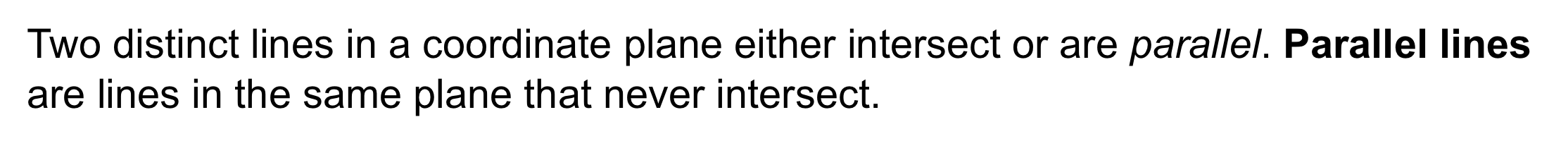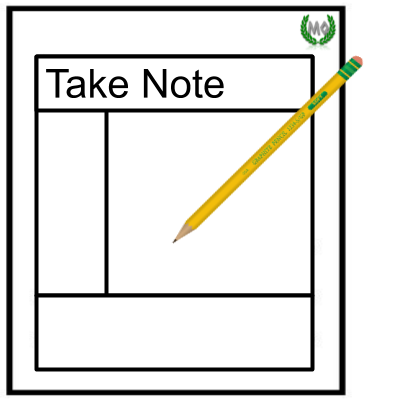2
3
4
2
10
Take Note: Define parallel lines.
3
10
Take note: If two lines are parallel, what do you know about their slopes ?
4
10
Take Note: Graph two lines that are parallel to each other. Zoom and pan your graph to establish an appropriate viewing window.5
5
10
Problem 1 Got It?
A
B
C
D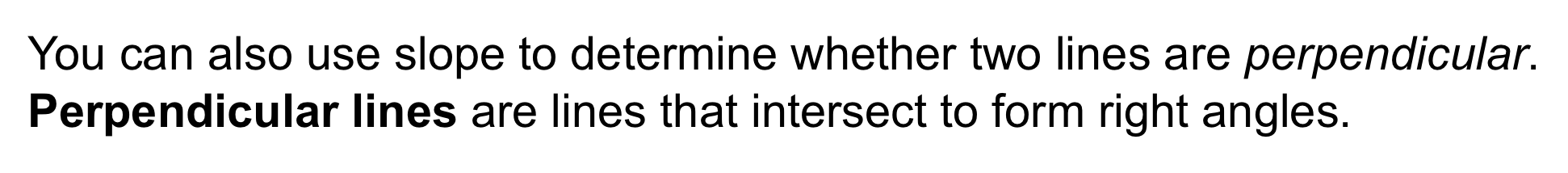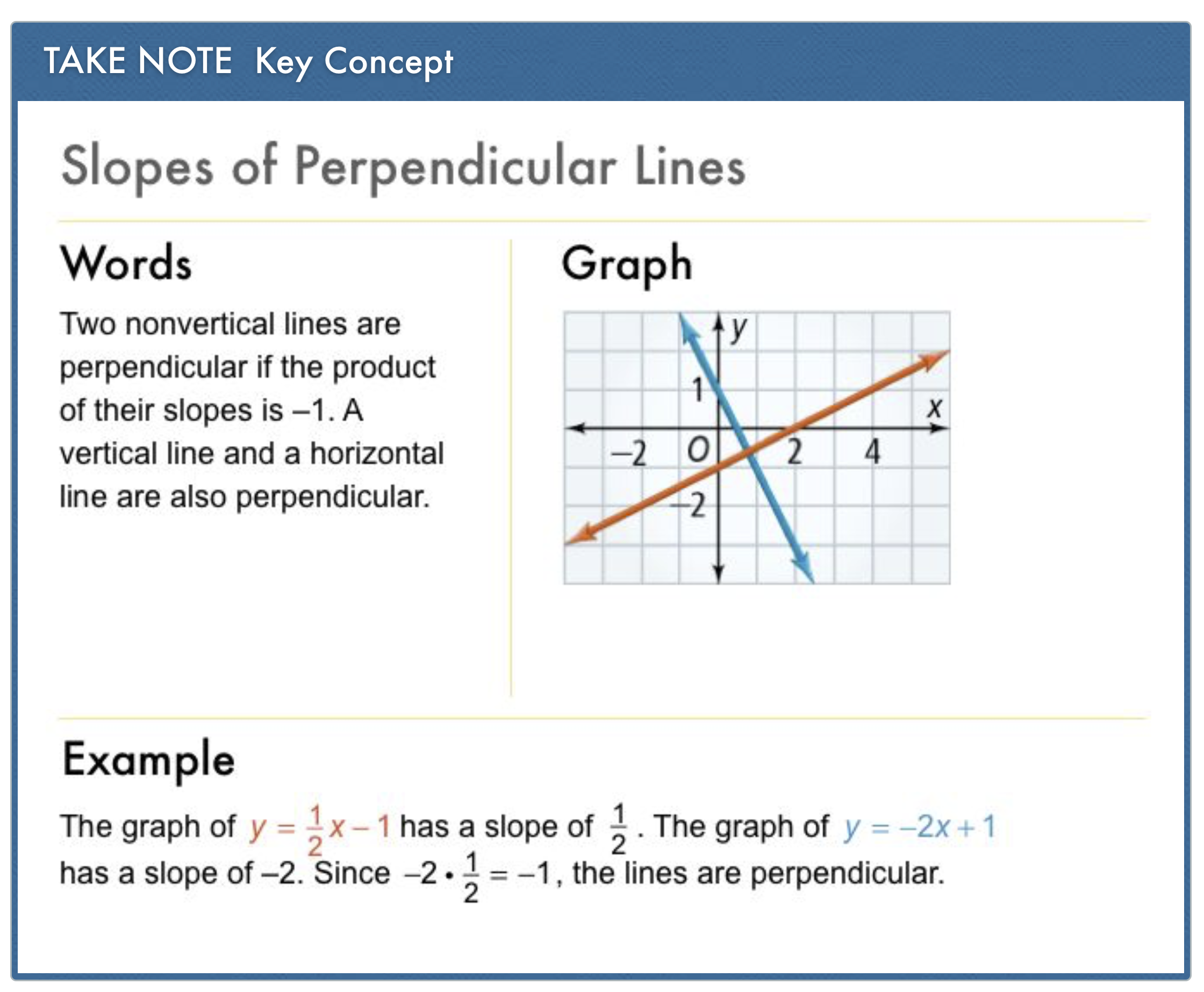6
7
8
9
6
10
Take Note: Define perpendicular lines.
7
10
Take note: If two lines are perpendicular, what do you know about their slopes ?
8
10
Take Note: Graph two lines that are perpendicular to each other. Zoom and pan your graph to establish an appropriate viewing window.
9
10
Take Note: Graph two lines that are neither parallel nor perpendicular to each other. Zoom and pan your graph to establish an appropriate viewing window.10
10
10
Problem 2 Got It?
A
B
C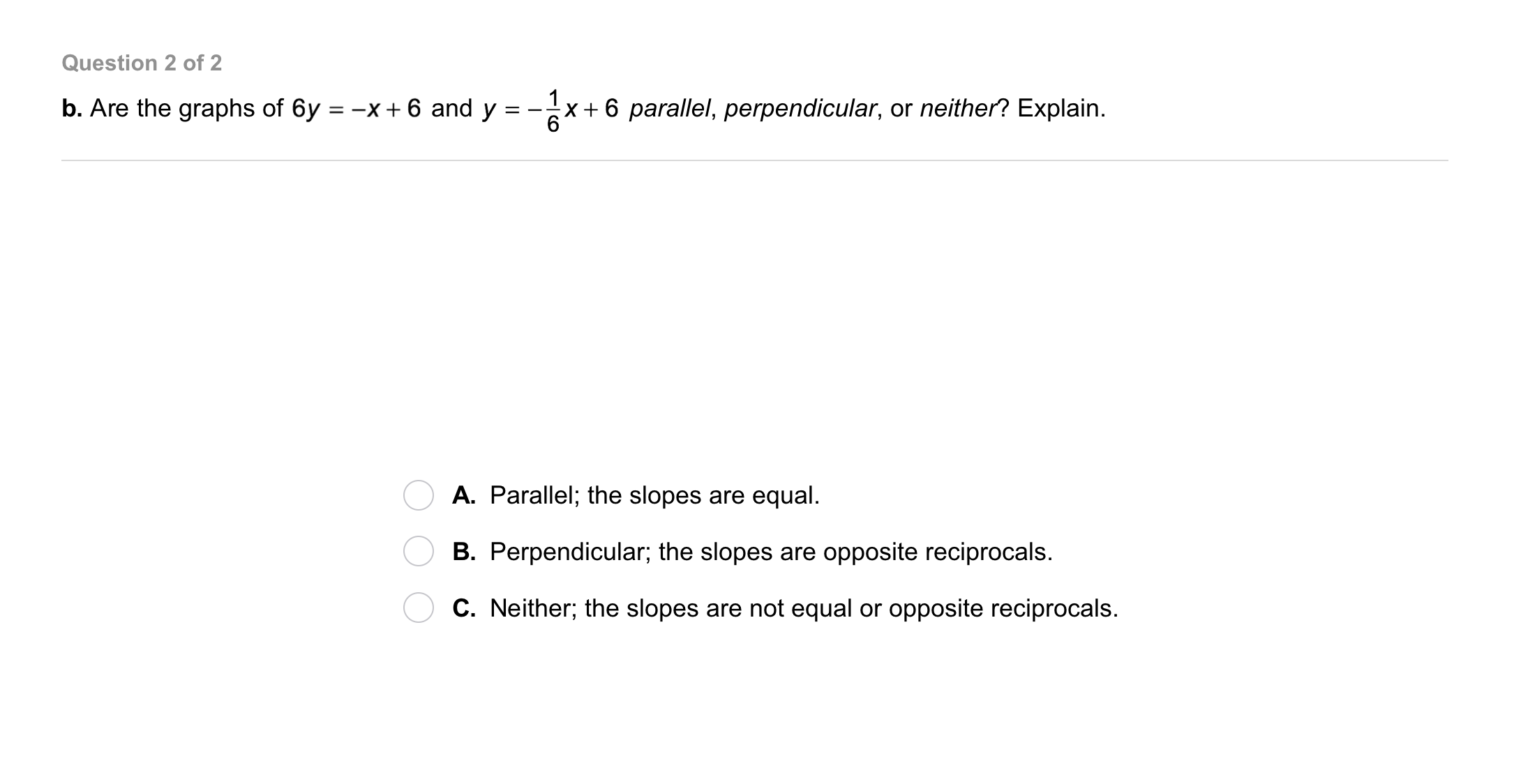11
11
10
Problem 2 Got It?
A
B
C12
12
10
Take Note: If you know the slope of a line and one point that the line includes, how can you write the equation of that line in point-slope form ?

You may reference the terms from the formula below in your response:13
13
10
Problem 3 Got It?
A
B
C
D14
14
10
Problem 4 Got It? What equation could the architect enter to represent a second beam whose graph will pass through the corner at (0, 10) and be parallel to the existing beam. An equation that represents the existing beam is:

A
B
C
D15
15
10
Take Note: Summarize the content of this lesson.